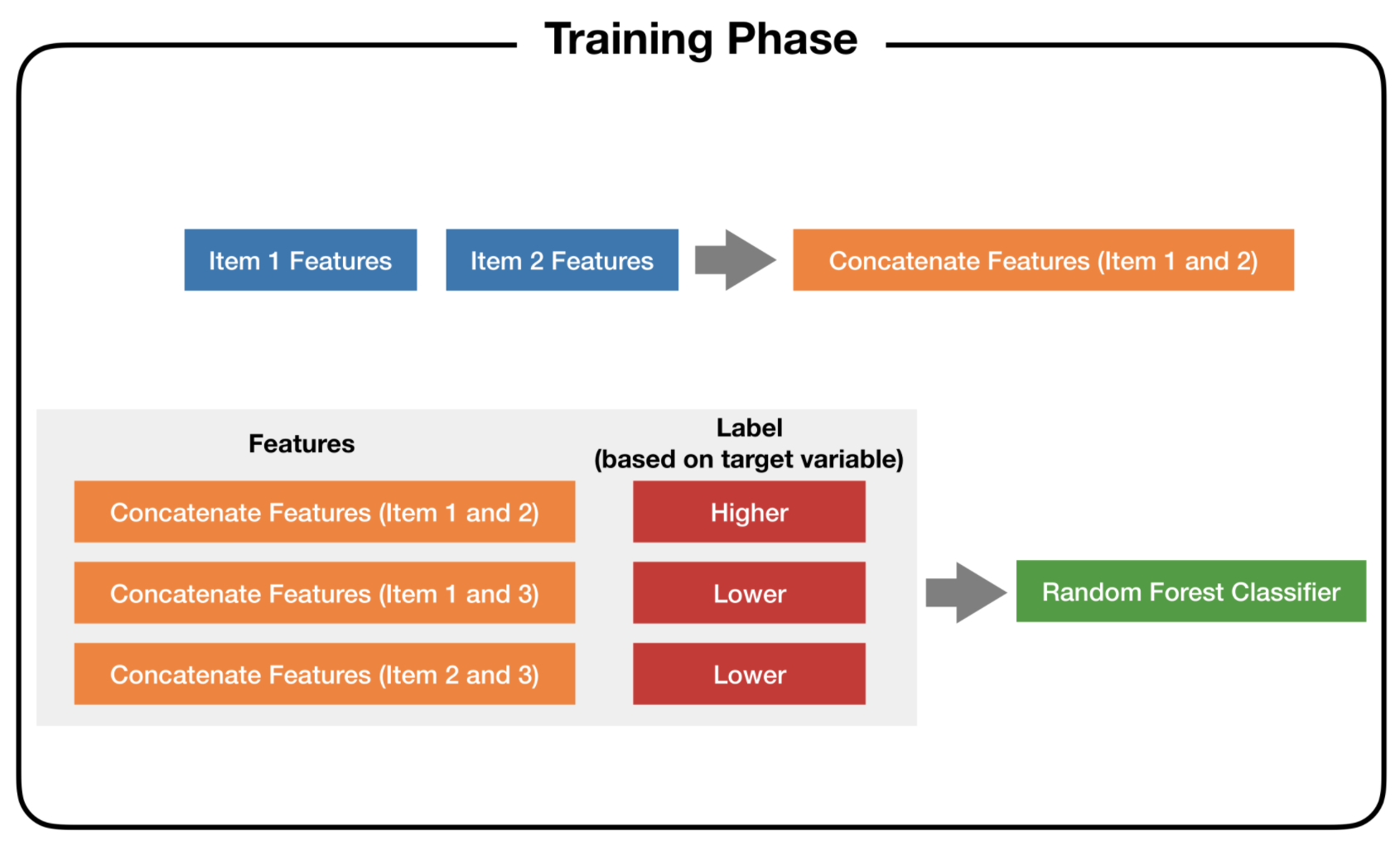# TIL: Learning to Place

Classification as a Heavy-Tail Regressor

Vincent Warmerdam koaning.io
2021-10-29

I haven’t benchmarked this idea, but it sounds like it might work.

## Heavy Tails

Let’s say that you want to have a regression algorithm on a dataset that has a large skew. Many values may be zero, but there’s a very long tail too. How might we go about regressing this?

We could … turn it into a classification problem instead.

## Classifier

Let’s say that we have an ordered dataset. Let’s say that item 1 has the smallest regression value and item $$n$$ has the highest value. That means that;

$y_1 \leq y_2 \leq ... \leq y_{n-1} \leq y_n$ Let’s now say we have a new datapoint $$y_{new}$$. Maybe we don’t need to perform regression. Maybe we just need to care about if $$y_{new} \leq y_1$$. If it is, we just predict $$y_{new} = y_1$$. If it’s not, we try $$y_1 \leq y_{new} \leq y_2$$. If that’s not it, we can try $$y_2 \leq y_{new} \leq y_3$$

This turns the problem on it’s head. We’re no longer worrying about how heavy the tail could be. Instead we’re wondering where in the order of our training data our new datapoint is. That means that we can use classification!Figure 1: The idea is to concatenate the features and to predict if the item is larger or smaller.

Given that we’ve trained a classifier that can be used to detect order, we can now use it as a heuristic to order new data.